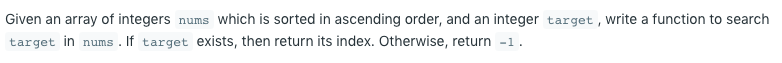## Binary Search in JavaScript

Binary Search is a computer science term used in programming and software engineering. It is a way of determining if a value exists in an ordered data set. It’s considered a textbook algorithm.

Imagine we are asked to search for an integer in an array that is sorted in ascending order. The challenge is to return the target number’s index.We could use a built in JavaScript function indexOf():

```var search = function(nums, target) {
var answer = nums.indexOf(target);
};
console.log(search([-1,0,3,4,5,9,12], 5)); // returns '4'
```

But that is an abstraction, which can be slow and expensive. Instead, we should implement our own binary search code.

## Binary Search Implementation

Binary search starts in the middle of a collection, and checks if that is the requested term. The initial middle position is determined by taking the length of the array and dividing it by two. If the array has an even number of elements, then the ‘halfway position’ is considered close enough. I use a JavaScript Math function to round down, making sure I am not left with a decimal floating number:

```cursor =  Math.floor( ((left + right) / 2) ); // about the middle
```

If the search term is not found, the position (the cursor) is moved. Since the collection is ordered from smallest to largest we know which direction to move the search cursor. If the target is less than the current value, we shift one to the left. If the target is greater than the current value, we shift two to the right.

This continues until the target query is found. That sequence is ran inside of a loop. The loop repeats while the left boundary is less than (or equal to) the right. The search cursor’s index is changed by adjusting the left or right bounds. Once adjusted, the cursor is recalculated on the subsequent iteration.

```var search = function(nums, target) {
var left = 0; // first element, initial left boundary
var right = nums.length;
right = right - 1; // last element, initial right boundary
var cursor = 0;
while(left <= right){
cursor =  Math.floor( ((left + right) / 2) ); // about the middle to start
console.log(cursor + ": " + nums[cursor]);
if(nums[cursor] === target){
return cursor; // query found!
}
if(target < nums[cursor]){
right = cursor - 1; // move cursor to the left by one
console.log("cursor moved to the left  by one")
}else{
left = cursor + 1; // move cursor to the right by two
console.log("cursor moved to the right by two")
}
}